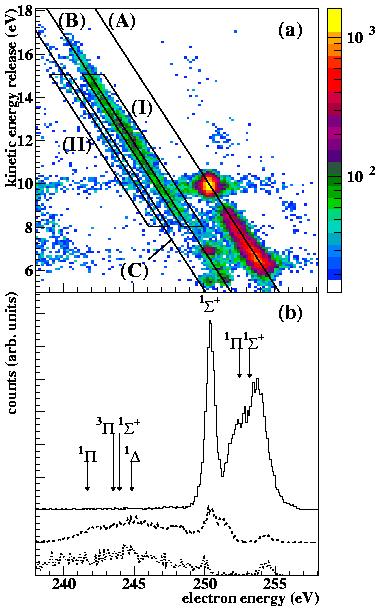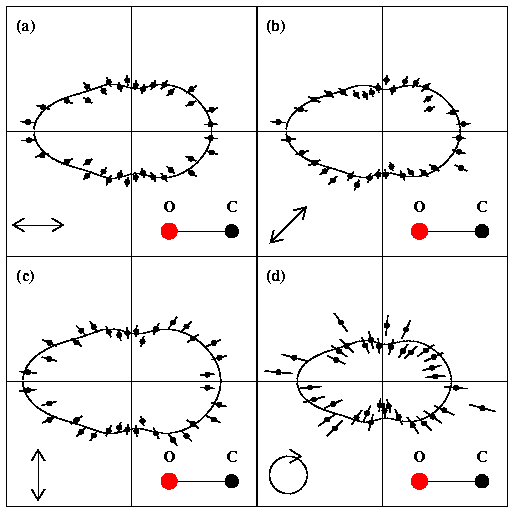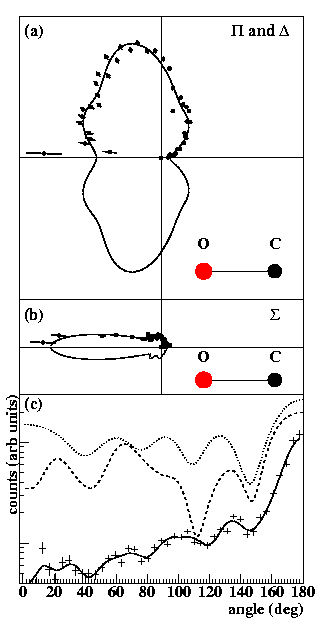### Auger Electron emission from Fixed-in-Space CO

 We have ejected a Carbon 1s electron from CO by absorption of 305eV photons and investigated the subsequent Auger decay of the K-hole. The key questions addressed by this study are: Can the creation of a core hole by photo-ionization (slow electron) and its subsequent Auger decay (fast electron) be treated as two independent steps? Disentangle the decay paths by determination of the final electronic state of the fragments Measure angular distribution of the Auger electrons in the body fixed frame of the molecule to search for diffraction effects. To answer these questions we have detected the Auger electron in coincidence with the charge state and momentum vector of the C+ and O+ fragments. The energy resolution for the Auger electrons is about 1 eV in the range investigated (see figure below). The experiment has been performed with linearly and circularly polarized photons. The linear polarization has been rotated from horizontal to vertical. The rotation has been monitored by the angular distribution of the C+ and O+ fragmentation axis for high kinetic energy release.Fig 1: Angular distribution of the C+ and O+ fragmentation axis while the polarization axis (indicated by the red arrow) of Bl. 4 at the Advanced Light Source is rotated in steps of 10 deg. From this we obtain a dataset which covers all direction of electron and molecular fragments with respect to the polarization axis. From the correlation between the Kinetic Energy Release and the Auger electron energy one knows the final electronic state of the C+ and O+ . This helps to conclude on the decay path in the correlation diagram.Auger decay of CO+ from 305 eV photon absorption leading to C+ + O+. a) horizontal axis Auger electron energy, vertical axis kinetic energy release. The diagonal lines correspond to decays of the CO+ (C(1s)-1) ground state to different final states of the ionic fragments: (A): C+(2P) + O+(4S) (ground state), (B): C+(2P) + O+(2D), (C): C+(2P) + O+(2P). b) Auger electron spectra corresponding to (A) (B) and (C) final state (from top to bottom). The arrows show the calculated mean energies for some states. These calculated transitions have a width of 3-5 eV. For some of these decay channel we have plotted the angular distribution of the Auger electrons. We find that: For all decay channels investigated the two step model holds: the observed angular distribution of the Auger electron in the molecular fixed frame does not depend on the polarization of the photon which creates the K-hole. The 1Sigma+ line at 250.5 eV (B-state) corresponds to a kintic energy release of 9-10 eV. For this decay channel the CO2+ molecule has time to rotate before fragmentation. Therefore the measured direction of fragmentation does not allow to "fix the molecule in space". For the decay indicated by region I and II in the above figure of KER versus electron energy the symmetry of the transition leaves strong traces in the angular distribution. The most pronounced effect is seen for region II which correspond to a S Auger electron. Here the Auger electron is emitted in a narrow "search light" cone in the direction of the Oxygen atom. On top of this a clear oscillatory pattern is found, which we trace back to a diffraction of the Auger electron wave in the two center potential.Angular distribution of Auger electrons from the narrow 1Sigma+ line at 250.5 eV (B-state). The orientation of the molecule is horizontal with the oxygen to the left as indicated. a-c linear polarized light, the polarization vector is indicated by the double arrow. (d) circular polarized light, propagating into the plane of the figure.Angular distribution of Auger electrons from CO+. (a) from region I Fig.1a, (b) region II Fig. 1a. (a) corresponds to the \$\rm CO+ (1Sigma+) -> CO2+ (1Delta, 3Pi, 1Pi) transition. b) corresponds to a CO+(1Sigma+) -> CO2+(1Sigma) transition. c) same data as in b) 0 deg corresponds to emission in direction of the carbon. The full lines in all figures are a fit of Legendre polynomials to guide the eye. Other lines in c) are results of a multiple scattering calculation for an S electron wave starting at the carbon center in CO2+. Dashed: both vacancies in the CO2+ at C(2p), dotted: one vacancy each at C(2p) and O(2p). The absolute height of the calculation is arbitrary. More detail can be found here: Auger electron emission from fixed in space CO Th. Weber, M. Weckenbrock, M. Balser, L. Schmidt, O. Jagutzki, W. Arnold, O. Hohn, E. Arenholz, T. Young, T. Osipov, L. Foucar, A. De Fanis, R. Diez Muino, H. Schmidt-Böcking, C. L. Cocke, M. H. Prior, and R. Dörner Phys.Rev.Lett., 90 (2003) 153003-1 Download this file here: Weber2003prl.pdf (251kb)     ZIP file (238kb)